In :
import numpy as np
import matplotlib.pyplot as plt
%matplotlib inline
from IPython.display import Image
import pandas as pd

Lecture 14:¶

• Learn how to plot histograms and cumulative distributions

• Learn how to get lists of random numbers

• Learn about the topography of the Earth (hypsometric curve)

The hypsometric curve for the Earth¶

The hypsometric curve for the Earth was very important in that it demonstrated that the Earth's crust comes in two flavors: continental and oceanic.

In :
Image(filename='Figures/hypsometric.v2_400.jpg')
Out: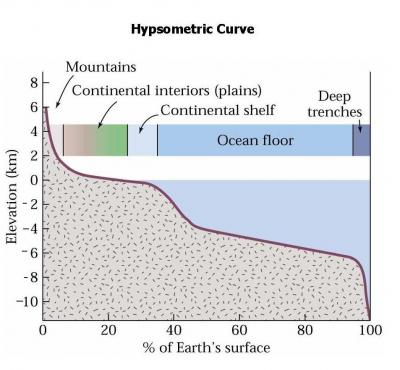Hypsometric curve for the Earth. The x-axis is the percentage of Earth's surface that falls above the elevation on the y-axis. For example, ~30% of the Earth's surface is above sea level. [Figure from this web site: https://serc.carleton.edu/mathyouneed/hypsometric/index.html ].

The insight from the hypsometric curve is that there are two plateaus in crustal elevations with the continents above sea level but another large fraction of crust below sea level. These are the two 'flavors' of continental and oceanic crust.

Oceanic crust must be denser than continental crust - that is why it "floats" lower which is the basis for isostacy. [For more on isostacy, see this link: https://en.wikipedia.org/wiki/Isostasy. ]

Today we will recreate a hypsometric from our knowledge of the Earth's topography. Nowadays, we can measure the topography from satellites, so there is extensive coverage over the surface of the Earth.

Here is a map projection of the Earth's topography. In the coming weeks, we will learn how to make this map, but for now, let's just take a look at it. Notice the mountain ranges, including the ridge crests.

In :
Image(filename='Figures/etopo20.jpg')
Out: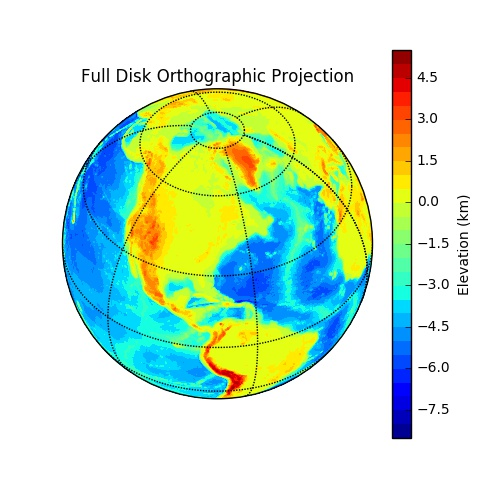We are interested in the hypsometric curve, which is the distribution of elevations. One very straight forward way to do this is to look at the elevation data as a histogram. A histogram breaks the data into 'bins', sums the data points within each bin and then plots the totals in each bin as a series of bars.

To plot a histogram, we must first read in the data that were plotted on the map. We'll use a set of elevation data associated with a latitude/longitude grid around the earth called ETOPO20 (20-minute resolution).

The file is a compressed version (gnu-zip or gz) of the data and can be read directly using NumPy's loadtxt( ) function:

In :

It is usually a good idea to take a peek at the data before you try plotting them, so let's just look at the first 10 cells in the array 'topo':

In :
print (topo[0:10])
[[2804.     2804.     2804.     ... 2804.     2804.     2804.    ]
[2831.     2831.     2831.     ... 2831.     2831.     2831.    ]
[2808.     2808.     2808.     ... 2808.     2808.     2808.    ]
...
[2899.     2899.     2899.     ... 2899.     2899.     2899.    ]
[2868.75   2868.75   2868.75   ... 2868.75   2868.75   2868.75  ]
[2785.5    2785.5    2795.3125 ... 2785.5    2785.5    2785.5   ]]

Our original hypsometric plot was in kilometers, whereas these data are in meters. We can convert the elevations to km by dividing topo by 1000 (because it is a NumPy array and not a list).

In :
topo_km=topo/1000. # convert to kilometers
print (topo_km[0:10])
[[2.804     2.804     2.804     ... 2.804     2.804     2.804    ]
[2.831     2.831     2.831     ... 2.831     2.831     2.831    ]
[2.808     2.808     2.808     ... 2.808     2.808     2.808    ]
...
[2.899     2.899     2.899     ... 2.899     2.899     2.899    ]
[2.86875   2.86875   2.86875   ... 2.86875   2.86875   2.86875  ]
[2.7855    2.7855    2.7953125 ... 2.7855    2.7855    2.7855   ]]

The elevations are in many rows that pair with the latitude and longitude data stored in two other files: etopo20lats.gz and etopo20lons.gz. For now, we're only concerned with the distribution of the topography, so we can "flatten" the data into a single row using the handy NumPy flatten( ) method you already met:

In :
flat_topo=topo_km.flatten()
print (flat_topo)
[ 2.804   2.804   2.804  ... -4.2915 -4.2915 -4.2915]

Now we can plot the data as a histogram using plt.hist( ). (Try help(plt.hist) for more info.)

plt.hist( ) takes some keyword arguments, and we will use bins and density to set the number of bins to 20 and normalize the plot to sum to unity.

In :
help(plt.hist)
Help on function hist in module matplotlib.pyplot:

hist(x, bins=None, range=None, density=None, weights=None, cumulative=False, bottom=None, histtype='bar', align='mid', orientation='vertical', rwidth=None, log=False, color=None, label=None, stacked=False, normed=None, hold=None, data=None, **kwargs)
Plot a histogram.

Compute and draw the histogram of *x*. The return value is a
tuple (*n*, *bins*, *patches*) or ([*n0*, *n1*, ...], *bins*,
[*patches0*, *patches1*,...]) if the input contains multiple
data.

Multiple data can be provided via *x* as a list of datasets
of potentially different length ([*x0*, *x1*, ...]), or as
a 2-D ndarray in which each column is a dataset.  Note that
the ndarray form is transposed relative to the list form.

Masked arrays are not supported at present.

Parameters
----------
x : (n,) array or sequence of (n,) arrays
Input values, this takes either a single array or a sequence of
arrays which are not required to be of the same length

bins : integer or sequence or 'auto', optional
If an integer is given, bins + 1 bin edges are calculated and
returned, consistent with :func:numpy.histogram.

If bins is a sequence, gives bin edges, including left edge of
first bin and right edge of last bin.  In this case, bins is
returned unmodified.

All but the last (righthand-most) bin is half-open.  In other
words, if bins is::

[1, 2, 3, 4]

then the first bin is [1, 2) (including 1, but excluding 2) and
the second [2, 3).  The last bin, however, is [3, 4], which
*includes* 4.

Unequally spaced bins are supported if *bins* is a sequence.

If Numpy 1.11 is installed, may also be 'auto'.

Default is taken from the rcParam hist.bins.

range : tuple or None, optional
The lower and upper range of the bins. Lower and upper outliers
are ignored. If not provided, *range* is (x.min(), x.max()).
Range has no effect if *bins* is a sequence.

If *bins* is a sequence or *range* is specified, autoscaling
is based on the specified bin range instead of the
range of x.

Default is None

density : boolean, optional
If True, the first element of the return tuple will
be the counts normalized to form a probability density, i.e.,
the area (or integral) under the histogram will sum to 1.
This is achieved by dividing the count by the number of
observations times the bin width and not dividing by the total
number of observations. If *stacked* is also True, the sum of
the histograms is normalized to 1.

Default is None for both *normed* and *density*. If either is
set, then that value will be used. If neither are set, then the
args will be treated as False.

If both *density* and *normed* are set an error is raised.

weights : (n, ) array_like or None, optional
An array of weights, of the same shape as *x*.  Each value in *x*
only contributes its associated weight towards the bin count
(instead of 1).  If *normed* or *density* is True,
the weights are normalized, so that the integral of the density
over the range remains 1.

Default is None

cumulative : boolean, optional
If True, then a histogram is computed where each bin gives the
counts in that bin plus all bins for smaller values. The last bin
gives the total number of datapoints. If *normed* or *density*
is also True then the histogram is normalized such that the
last bin equals 1. If *cumulative* evaluates to less than 0
(e.g., -1), the direction of accumulation is reversed.
In this case, if *normed* and/or *density* is also True, then
the histogram is normalized such that the first bin equals 1.

Default is False

bottom : array_like, scalar, or None
Location of the bottom baseline of each bin.  If a scalar,
the base line for each bin is shifted by the same amount.
If an array, each bin is shifted independently and the length
of bottom must match the number of bins.  If None, defaults to 0.

Default is None

histtype : {'bar', 'barstacked', 'step',  'stepfilled'}, optional
The type of histogram to draw.

- 'bar' is a traditional bar-type histogram.  If multiple data
are given the bars are arranged side by side.

- 'barstacked' is a bar-type histogram where multiple
data are stacked on top of each other.

- 'step' generates a lineplot that is by default
unfilled.

- 'stepfilled' generates a lineplot that is by default
filled.

Default is 'bar'

align : {'left', 'mid', 'right'}, optional
Controls how the histogram is plotted.

- 'left': bars are centered on the left bin edges.

- 'mid': bars are centered between the bin edges.

- 'right': bars are centered on the right bin edges.

Default is 'mid'

orientation : {'horizontal', 'vertical'}, optional
If 'horizontal', ~matplotlib.pyplot.barh will be used for
bar-type histograms and the *bottom* kwarg will be the left edges.

rwidth : scalar or None, optional
The relative width of the bars as a fraction of the bin width.  If
None, automatically compute the width.

Ignored if *histtype* is 'step' or 'stepfilled'.

Default is None

log : boolean, optional
If True, the histogram axis will be set to a log scale. If
*log* is True and *x* is a 1D array, empty bins will be
filtered out and only the non-empty (n, bins, patches)
will be returned.

Default is False

color : color or array_like of colors or None, optional
Color spec or sequence of color specs, one per dataset.  Default
(None) uses the standard line color sequence.

Default is None

label : string or None, optional
String, or sequence of strings to match multiple datasets.  Bar
charts yield multiple patches per dataset, but only the first gets
the label, so that the legend command will work as expected.

default is None

stacked : boolean, optional
If True, multiple data are stacked on top of each other If
False multiple data are arranged side by side if histtype is
'bar' or on top of each other if histtype is 'step'

Default is False

normed : bool, optional
Deprecated; use the density keyword argument instead.

Returns
-------
n : array or list of arrays
The values of the histogram bins. See *normed* or *density*
and *weights* for a description of the possible semantics.
If input *x* is an array, then this is an array of length
*nbins*. If input is a sequence arrays
[data1, data2,..], then this is a list of arrays with
the values of the histograms for each of the arrays in the
same order.

bins : array
The edges of the bins. Length nbins + 1 (nbins left edges and right
edge of last bin).  Always a single array even when multiple data
sets are passed in.

patches : list or list of lists
Silent list of individual patches used to create the histogram
or list of such list if multiple input datasets.

Other Parameters
----------------
**kwargs : ~matplotlib.patches.Patch properties

--------
hist2d : 2D histograms

Notes
-----
.. [Notes section required for data comment. See #10189.]

.. note::
In addition to the above described arguments, this function can take a
**data** keyword argument. If such a **data** argument is given, the
following arguments are replaced by **data[<arg>]**:

* All arguments with the following names: 'weights', 'x'.

In :
plt.hist(flat_topo,bins=20,density=True)
plt.xlabel("Elevation (km)")
plt.ylabel("Fraction");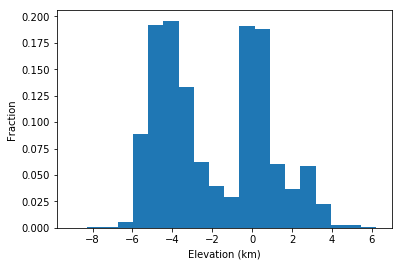There are two peaks, one near sea-level (the continents) and one below sea-level (the ocean basins). We saw this same pattern in the hypsometric curve.

But the hypsometric curve is not a histogram. It is in fact a kind of cumulative distribution function (CDF). Instead of binning the data and finding the number of points in each bin as in a histogram, we find the cumulative distribution for each value of x. We plot on the y axis the proportion of values less than or equal to x for each x. So the value of y on the hypsometric curve represents the percentage of Earth's surface with an elevation LOWER than the elevation at the corresponding value of x.

Let's create a CDF from our topographic data. To do this, we must sort the data by elevation, then plot each elevation against a number from 1 to 100%.
To sort the data, we can use the sorted( ) function on our flat_topo array. (This is similar to, but has a different syntax from, the way we sorted Panda DataFrames.)

In :
topo_sorted=sorted(flat_topo) # sort the array in increasing numbers

For the y axis we need the percentage of Earth's surface that lies below a given elevation. For this, we just need an array with $N$ values equally spaced between 0 and 100. This sounds like a job for np.linspace( ) [Lecture 7].

In :
percent=np.linspace(0,100,len(topo_sorted))

And now for the plot:

In :
plt.plot(topo_sorted,percent)
plt.xlabel('Elevation (km)')
plt.ylabel('Percent');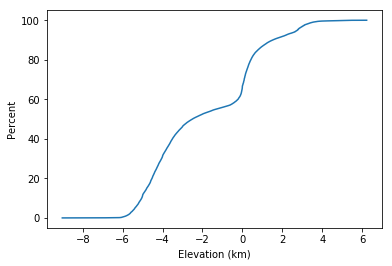Compare this plot with the histogram. The same two peaks from the histogram correspond to the abrupt increases in percent values at ~-4 km and ~0 km. The advantage of a CDF over a histogram is that you don't have to choose some arbitrary bin size.

If you look closer, you can see that this CDF is similar to our hypsometric curve but turned on its side and flipped left to right. Once we plot the data with the percent on the x-axis and the elevation on the y-axis, we'll get closer to our hypsometric curve:

In :
plt.plot(percent,topo_sorted)
plt.xlabel('Percent')
plt.ylabel('Elevation (km)');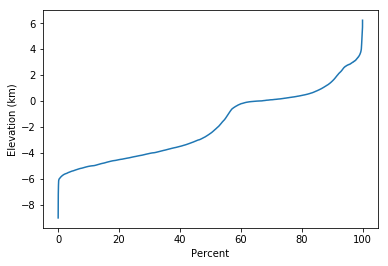Our CDF is still different from the hypsometric curve we saw at the beginning of class - it is more like the mirror image of what we are after.

In :
Image(filename='Figures/hypsometric.v2_400.jpg')
Out:Our CDF represents the percentage (x-axis) of Earth's surface that is LOWER than the corresponding elevation (y-axis), whereas the hypsometric curve represents the percentage of Earth's surface HIGHER than the corresponding elevation. So let's start over and sort the data by decreasing values instead of increasing. We do that with the keyword argument reverse in the function sorted( ).

In :
topo_sorted=sorted(flat_topo,reverse=True) # sort the data by decreasing numbers
percent=np.linspace(0,100,len(topo_sorted)) # and percent just like before
plt.plot(percent,topo_sorted) # plot
plt.xlabel('Percent')  # label
plt.ylabel('Elevation (km)');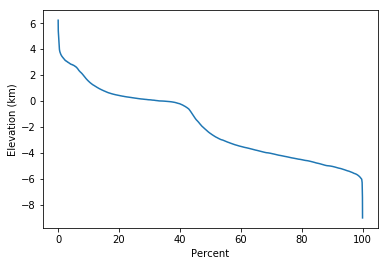NOW we are getting somewhere! But, the dimensions of the plot are different. We can change this by using the plt.figure( ) function, which as we already learned takes a tuple of the desired dimensions and a figure number as arguments. Here we make a 6 x 5 figure.

In :
plt.figure(figsize=(6,5)) #figure number and dimensions
plt.plot(percent,topo_sorted)
plt.ylabel('Elevation (km)')
plt.title("Hypsometric Curve")
plt.xlabel("Percent");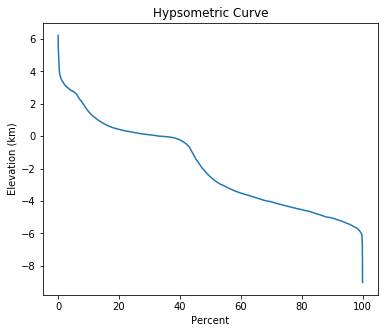We're close but still not exactly right.

Recall that the data are binned into 20 minute grids of latitude and longitude. The problem with that approach is illustrated in the figure below. On the left is the way our data are constructed with equal weight at every line of latitude. On the right I replotted the data as a polar projection, with the North pole in the center. And yes you will learn how to do that too! But for now, you can see that there is a lot less surface area per line of latitude near the north pole than at the equator!

In :
Image(filename='Figures/etopo20_polar.jpg')
Out: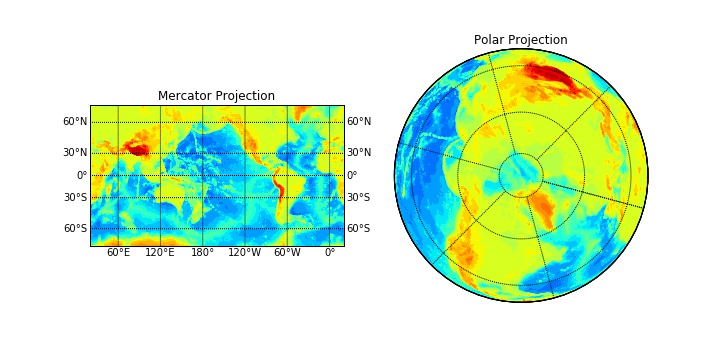Because the area of one cell at the pole is MUCH less than that for an equatorial cell, we have to normalize the number of data points in each cell by its surface area. We can accomplish the same thing by re-sampling each grid and not use ALL the data but just select a number that is proportional to the surface area it covers. Each point would then represent the same percentage of Earth's surface. We would sample the most (say take all the grid cells) from the equator and few as we get to the poles.

To do this, we'll need to know how many cells are in each latitudinal bin. Let's use the NumPy shape attribute for that:

In :
topo.shape
Out:
(540, 1081)

This means that there are 540 latitudinal bins with 1081 longitudinal cells in each latitude bin.

Let's read in the latitude/longitude data files that defines our grid and take a look at them.

In :
print (lats[0:20])
print (lons[0:20])
print (lats.shape)
print (lons.shape)
[-89.8333333 -89.5       -89.1666667 -88.8333334 -88.5000001 -88.1666668
-87.8333335 -87.5000002 -87.1666669 -86.8333336 -86.5000003 -86.166667
-85.8333337 -85.5000004 -85.1666671 -84.8333338 -84.5000005 -84.1666672
-83.8333339 -83.5000006]
[20.1666667 20.5       20.8333333 21.1666666 21.4999999 21.8333332
22.1666665 22.4999998 22.8333331 23.1666664 23.4999997 23.833333
24.1666663 24.4999996 24.8333329 25.1666662 25.4999995 25.8333328
26.1666661 26.4999994]
(540,)
(1081,)

There are 540 latitudinal bins and for each latitude and 1081 longitudinal bins.
The trick here is that the surface area $S$ of a lat/lon box varies as the cosine of the latitude ($\phi$ - see coordinate system in the figure below). In fact:

$$S = \int_{\phi_1}^{\phi_2} R\bigl( \int_{\lambda_1}^{\lambda_2} R \cos \phi d\lambda\bigr) d\phi.$$
In :
Image(filename='Figures/Sphere_wireframe.png',width=400)
Out: